# Rotations of order 4 and reflections

The remaining patterns are numbered 1184, which implies that one more further question needs to the answered.

The first group are examples of patterns whose angles are all multiples of 90 degrees. An example of two of these are: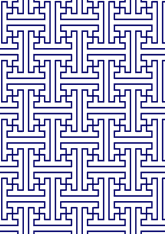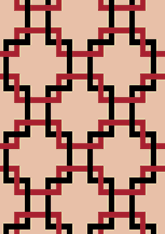The number in this group is 56, which is 4% of the total. Another question is needed - for this see page.
The second group are examples of patterns containing a regular 8-pointed star whose vertex angle is 45 or 90 degrees (as illustrated below). An example of two of these are: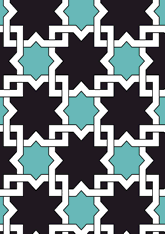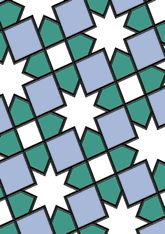The number in this group is 565, which is 47% of the total. Another question is needed - for this see page.
The third group are examples of patterns whose angles are all multiples of 45 degrees but does not include the two stars in the previous selection. An example of two of these are: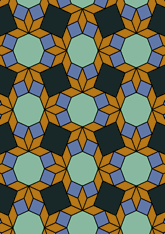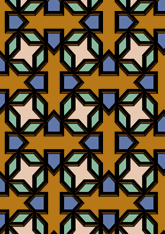The number in this group is 231, which is 19% of the total. Another question is needed - for this see page.
The fourth group are examples of patterns which contain a 6-pointed or 12-pointed star. An example of two of these are: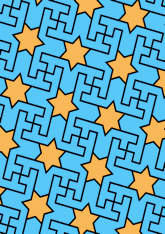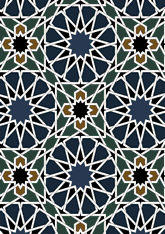The number in this group is 121, which is 10% of the total. Another question is needed - for this see page.
The last group are examples of patterns is none of the above. An example of two of these are: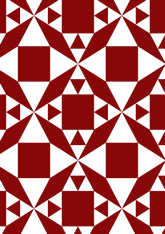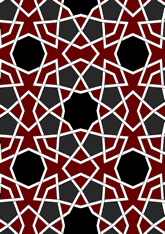The number in this group is 211, which is 17% of the total. Another question is needed - for this see page.

v53

Tiling Search was created by Brian Wichmann, and is maintained by MIT Libraries, through support from the Aga Khan Documentation Center.

Also supported by Performant Software Solutions LLC.